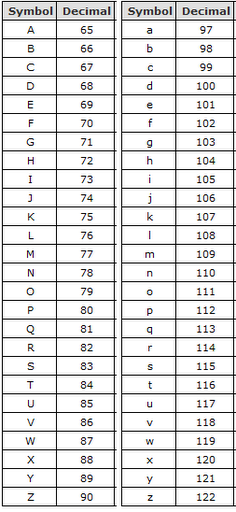# Introduction

As we have already seen, a Variable is an identifier which points to a value of a certain data type stored at a specific location in memory. The reason a variable needs to be associated with a specific data type is so that the computer knows how to interpret the binary data stored in memory. So, for example, 1000001 could either be the number 65 or the character "A". The only way the computer will know how to interpret this binary data is by the data type associated with the variable pointing to that value in memory.

The In this section we will learn more about data types, their uses, and see some examples.

# Categories of Data Types

There are three main categories of Data Types:

1. Primitive: Primitive data types cannot be broken down into simpler data types. They consist of a single value, accessible from a single address in memory.
2. Composite: Composite data types are made up of Primitive data types. They consist of multiple values at multiple addresses in memory.
3. Abstract Data Types (ADT): These are mathematical models for certain classs of data structures. This is outside of the scope of this course, but for those interested in reading more about this class of data types, take a look at the Wikipedia entry found at http://en.wikipedia.org/wiki/Abstract_data_type

## Primitive Data Types

For this section, we will focus on Primitive data types, and in the following section we will take a look at an example of a Composite data type, called an Array. Let's now look at some common Primitive Data Types.

### Boolean (bool)

A boolean value is the simplest type of data. It is simply either a 1 or a 0. In many programming languages, boolean values are made use of by the labels "true" and "false"; where "true" corresponds to 1, and "false" corresponds to 0.

In Python, we can create boolean variables as follows:

``````a = True
b = False
``````

To switch a true to a false, or a false to a true, we can use the "not" operator. To experiment with this, open up the Python interactive interpreter and follow along:

``````happy = True
print happy
[Output: True]
unhappy = not happy
print unhappy
[Output: False]
``````

### Integer (int)

A very common data type you will come across is the Integer. "Integer" is a class of numbers, also referred to as "whole numbers"; so any number without a fraction or decimal point. Integers range from 0 upwards, and also include negative numbers.

Examples of Integers are: -1000, -45, -2, 0, 13, 48, 600, 3000

To create variables in Python of type integer, we simply assign an integer value to a variable.

``````x = 10
print x
[Output: 10]
type(x)
[Output: ]
``````

Integers are very useful for counting things and for storing information about us: Our age, number of siblings, how many times have I visited P2PU today, and so on.

### Character (char)

A character is simply a letter of the alphabet, so from A-Z or a-z. Like all other data types, character data is stored as binary data in memory, which means that at some point the character will need to be converted into a number representation. The exact number that is assigned to each character is dependent on a specific encoding scheme that is being used. A very well known encoding is called ASCII (pronounced "ass-key" [ok, you can stop laughing]). Here is the ascii table, showing the integer associations to characters:To create a variable in Python that stores a character, simply assign a character value to a variable.

``````category = "A"
print category
[Output: A]
``````

### String (str)

Strings are another very common and useful data type. Strings are called strings because they are a "string of characters" (imagine characters all strung together to form a sentence). Strings are useful for any text data, from a single word, to a couple of paragraphs.

Important to remember: When dealing with strings and characters in Python (and most languages), they need to be surrounded by double inverted commas (" <- this). The reason for this is so that the programming language interpreter can differentiate between code and string values.

To create a string variable in Python, simply wrap the string in double inverted commas, and assign it to a variable.

``````my_string = "This is my string"
print my_string
[Output: This is my string]
``````

### Float (float)

Sometimes, we need to deal with numbers which have a fraction or decimal component. This is very common, for example, with currency. For example, if I need to store the price of eggs as 50 cents, the numerical value would be 0.5 . Because this has a decimal point, we cannot use an Integer data type. This is where the Float data type comes in. The reason it is called "float" is because the method of approximating real numbers is called "Floating Point".

Floats are useful for currency, as well as for mathematical calculations which require the precision of the decimal point. For example:

``````price = 13.50
print price
[Output: 13.5]
type(price)
[Output: ]
``````

# Exercises

1. Create two variables, one with the value of 50, and the other with the value of 1.5 . Investigate the data types of each of these two values with the type() function. Then create a third variable which is the sum of the first two variables. What is the data type of this new variable?
2. What happens when you try and add a String to an Integer?
3. Try writing your name as ASCII integer values.

Continue to next section